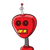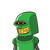# 4.Sum of the zeroes of the polynomial p(x) = x2 – kx + 9 is 7. Find thevalue of K​

4.
Sum of the zeroes of the polynomial p(x) = x2 – kx + 9 is 7. Find the
value of K

### 2 thoughts on “4.<br />Sum of the zeroes of the polynomial p(x) = x2 – kx + 9 is 7. Find the<br />value of K<br />​”

1.Step-by-step explanation:

x² – kx + 9

sum of zeros

=-(b/a)

=-(-k/1)=7

So k=7

2.